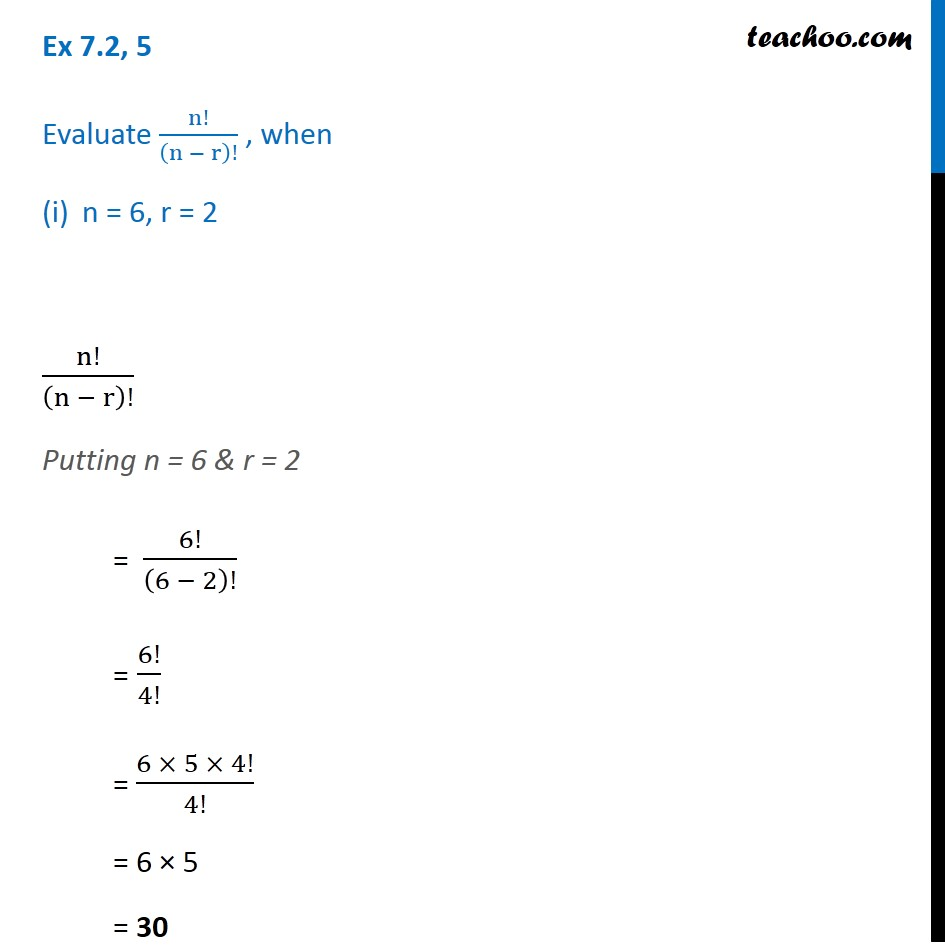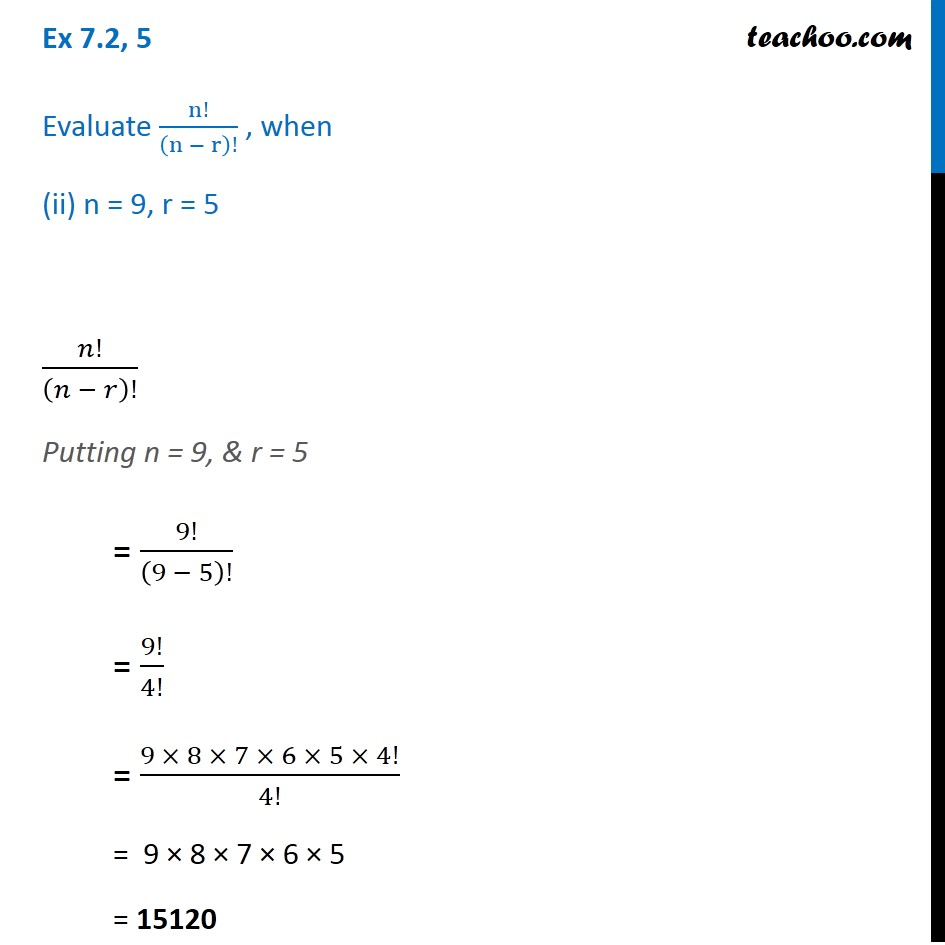Subscribe to our Youtube Channel - https://you.tube/teachoo

1. Chapter 7 Class 11 Permutations and Combinations
2. Serial order wise
3. Ex 7.2

Transcript

Ex 7.2, 5 Evaluate n!/(n − r)! , when n = 6, r = 2 n!/(n − r)! Putting n = 6 & r = 2 = 6!/(6 − 2)! = 6!/4! = (6 × 5 × 4!)/4! = 6 × 5 = 30 Ex 7.2, 5 Evaluate n!/(n − r)! , when (ii) n = 9, r = 5 𝑛!/(𝑛 − 𝑟)! Putting n = 9, & r = 5 = 9!/(9 − 5)! = 9!/4! = (9 × 8 × 7 × 6 × 5 × 4!)/4! = 9 × 8 × 7 × 6 × 5 = 15120 Ex 7.2, 5 Evaluate n!/(n − r)! , when (ii) n = 9, r = 5 𝑛!/(𝑛 − 𝑟)! Putting n = 9, & r = 5 = 9!/(9 − 5)! = 9!/4! = (9 × 8 × 7 × 6 × 5 × 4!)/4! = 9 × 8 × 7 × 6 × 5 = 15120

Ex 7.2